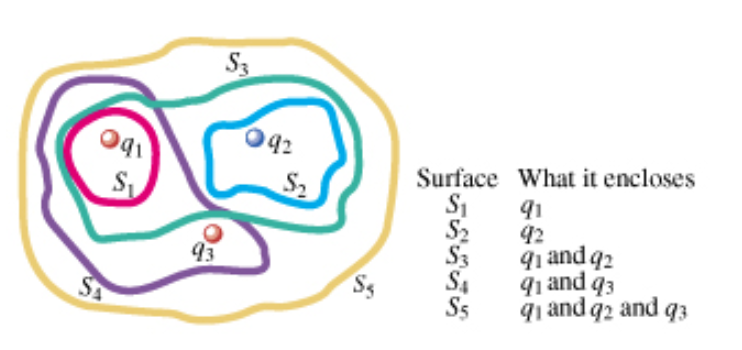# Problem: The three small spheres shown in the figure (Figure 1) carry charges q1 = 4.35nC , q2 = -8.00nC , and q3= 2.25nC.Find the net electric flux through the closed surface S1 shown in cross-section in the figure.

###### FREE Expert Solution

Gauss law:

$\overline{){{\mathbf{\varphi }}}_{{\mathbf{E}}}{\mathbf{=}}\frac{{\mathbf{q}}_{\mathbf{e}\mathbf{n}\mathbf{c}}}{{\mathbf{\epsilon }}_{\mathbf{0}}}}$

97% (365 ratings)###### Problem Details

The three small spheres shown in the figure (Figure 1) carry charges q1 = 4.35nC , q2 = -8.00nC , and q3= 2.25nC.

Find the net electric flux through the closed surface S1 shown in cross-section in the figure.